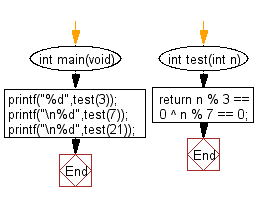﻿ C : Check if a non-ve is a multiple of 3 or 7, but not both# C Exercises: Check whether a non-negative given number is a multiple of 3 or 7, but not both

## C-programming basic algorithm: Exercise-20 with Solution

Write a C program to check whether a non-negative given number is a multiple of 3 or 7, but not both.

C Code:

``````#include <stdio.h>
#include <stdlib.h>

int main(void){
printf("%d",test(3));
printf("\n%d",test(7));
printf("\n%d",test(21));
}

int test(int n)
{
return n % 3 == 0 ^ n % 7 == 0;

}
``````

Sample Output:

```1
1
0
```

Pictorial Presentation:Flowchart:C Programming Code Editor:

What is the difficulty level of this exercise?

Test your Programming skills with w3resource's quiz.

﻿

## C Programming: Tips of the Day

Why doesn't a+++++b work?

printf("%d",a+++++b); is interpreted as (a++)++ + b according to the Maximal Munch Rule!.

++ (postfix) doesn't evaluate to an lvalue but it requires its operand to be an lvalue.

! 6.4/4 says the next preprocessing token is the longest sequence of characters that could constitute a preprocessing token"

Ref : https://bit.ly/3fdldUT